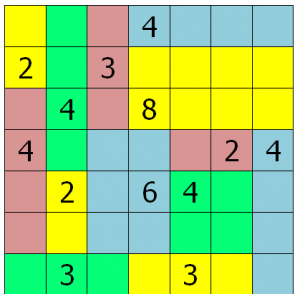# RectanglesA solved puzzle

Can you figure out the rules of the game from its solution?

Rectangles  connects arithmetic and geometry.  For students who have already started multiplication and division this puzzle makes factoring tangible.  For students who are just learning about multiplication, the puzzle provides a concrete introduction to multiplication as repeated addition.

Find more puzzles for younger students at Math in English, where they are called Shikaku.

Find many more puzzles in different sizes at Simon Tatham’s site.

Here are 30 pages worth of Rectangles puzzles generated from Simon Tatham’s code!